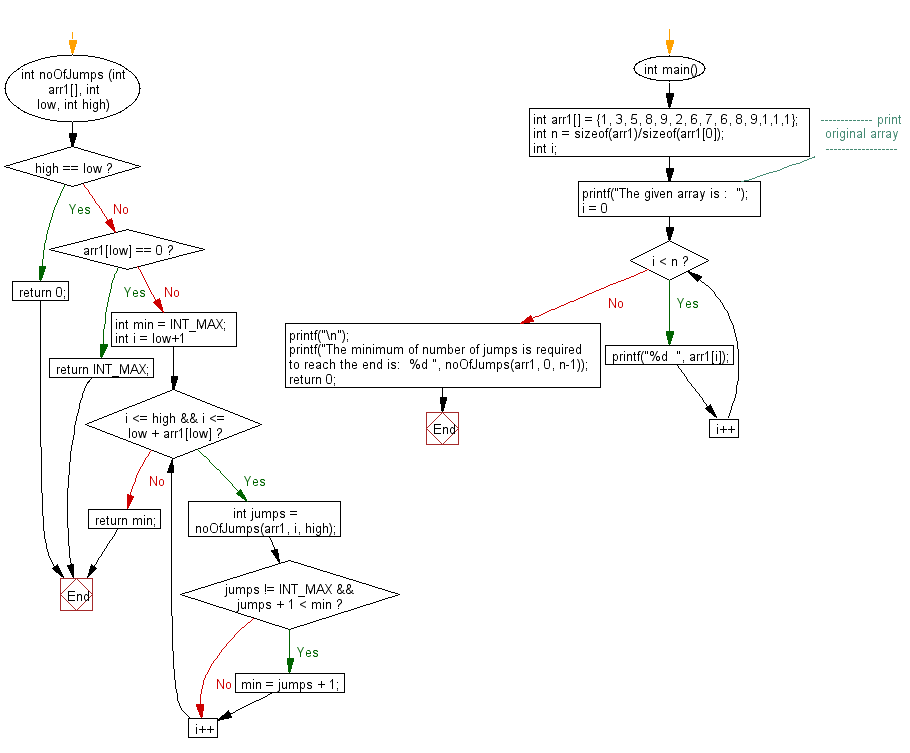﻿ C exercises: Return the minimum number of jumps to reach the end of the array - w3resource# C Exercises: Return the minimum number of jumps to reach the end of the array

## C Array: Exercise-56 with Solution

Write a program in C to return the minimum number of jumps to reach the end of the array.

Sample Solution:

C Code:

``````#include <stdio.h>
#include <limits.h>

int noOfJumps (int arr1[], int low, int high)
{
if (high == low)
return 0;
if (arr1[low] == 0)
return INT_MAX;
int min = INT_MAX;
for (int i = low+1; i <= high && i <= low + arr1[low]; i++)
{
int jumps = noOfJumps(arr1, i, high);
if(jumps != INT_MAX && jumps + 1 < min)
min = jumps + 1;
}
return min;
}

int main()
{
int arr1[] = {1, 3, 5, 8, 9, 2, 6, 7, 6, 8, 9,1,1,1};

int n = sizeof(arr1)/sizeof(arr1);
int i;
//------------- print original array ------------------
printf("The given array is :  ");
for(i = 0; i < n; i++)
{
printf("%d  ", arr1[i]);
}
printf("\n");
//------------------------------------------------------
printf("The minimum of number of jumps is required to reach the end is:  %d ", noOfJumps(arr1, 0, n-1));
return 0;
}
```
```

Sample Output:

```The given array is :  1  3  5  8  9  2  6  7  6  8  9  1  1  1
The minimum of number of jumps is required to reach the end is:  3
```

Flowchart:C Programming Code Editor:

Improve this sample solution and post your code through Disqus.

What is the difficulty level of this exercise?

﻿

## C Programming: Tips of the Day

Where is the C auto keyword used?

auto is a modifier like static. It defines the storage class of a variable. However, since the default for local variables is auto, you don't normally need to manually specify it.

Ref : https://bit.ly/3yzwC9r